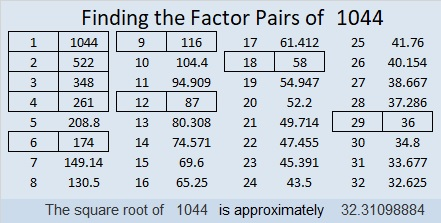# 1044 and Level 1

All of the clues in this puzzle have three common factors, but only one of those three factors won’t put a number greater than twelve in either the first column or the top row. Can you figure out what that common factor is as well as all the other factors that belong in this puzzle?Now I’ll share some information about the number 1044:

• 1044 is a composite number.
• Prime factorization: 1044 = 2 × 2 × 3 × 3 × 29, which can be written 1044 = 2² × 3² × 29
• The exponents in the prime factorization are 2, 2 and 1. Adding one to each and multiplying we get (2 + 1)(2 + 1)(1 + 1) = 3 × 3 × 2 = 18. Therefore 1044 has exactly 18 factors.
• Factors of 1044: 1, 2, 3, 4, 6, 9, 12, 18, 29, 36, 58, 87, 116, 174, 261, 348, 522, 1044
• Factor pairs: 1044 = 1 × 1044, 2 × 522, 3 × 348, 4 × 261, 6 × 174, 9 × 116, 12 × 87, 18 × 58 or 29 × 36
• Taking the factor pair with the largest square number factor, we get √1044 = (√36)(√29) = 6√29 ≈ 32.3109930² + 12² =1044

1044 is the hypotenuse of a Pythagorean triple:
720-756-1044 calculated from 2(30)(12), 30² – 12², 30² + 12².
It is also (20-21-29) times 36.

1044 is the sum of twin primes: 521 + 523 = 1044

1044 looks interesting a few other bases:
It’s 414 in BASE 16 because 4(16²) + 1(16) + 4(1) = 1044,
TT in BASE 35 (T is 29 base 10) because 29(35) + 29(1) = 29(35 + 1) = 29(36) = 1044, and T0 in BASE 36 because 29(36) = 1044

This site uses Akismet to reduce spam. Learn how your comment data is processed.